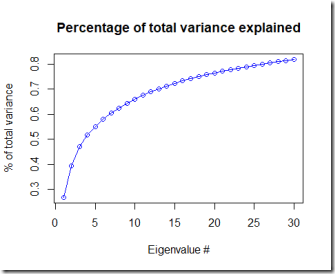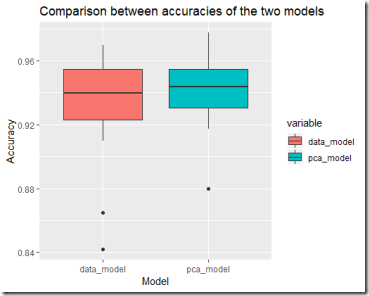Want to share your content on R-bloggers? click here if you have a blog, or here if you don't.

This is a short post following the previous one (PCA revisited).

In this post I’m going to apply PCA to a toy problem: the classification of faces. Again I’ll be working on the Olivetti faces dataset. Please visit the previous post PCA revisited to read how to download it.

The goal of this post is to fit a simple classification model to predict, given an image, the label to which it belongs. I’m goint to fit two support vector machine models and then compare their accuracy.

The

1. The first model uses as input data the raw (scaled) pixels (all 4096 of them). Let’s call this model “the data model”.
2. The second model uses as input data only some principal components. Let’s call this model “the PCA model”.

This will not be an in-depth and detailed fit and subsequent evaluation of the model, but rather a simple proof of concept to prove that a limited number of principal components can be used to perform a classification task instead of using the actual data.

## The setup of the two models

In order to get an idea of what accuracy our models can achieve, I decided to do a simple 20 fold crossvalidation  of each model.

The data model is a simple support vector machine that is fitted using all the faces in the training set: it’s input data is a vector of 4096 components.

The PCA model is again the same support vector machine (with the same hyperparameters, which however may need some tweaking) fitted using 30 PCs.

By performing PCA on the dataset I transformed the data and, according to the analysis, 30 PCs account for about 82% of the total variance in the dataset. Indeed you can see from the plots below that the first 30 eigenvalues are those with the highest magnitude.## Results

After running  the two crossvalidations on the two different models, the main results I found are the following:

1. The average accuracy of the two models is about the same (PCA model has a slightly better average accuracy): 93% vs 94%. Standard deviation seems to be again in favour of the PCA model: 3.2% vs 2.3%. The accuracy isn’t great, I obtained 98% on this dataset using other models, but of course this is just a test “out of the box” with no hyperparameter tuning and all the other fine tuning operations.
2. The second model fits the data much faster than the first one.

The results are summarised in the boxplot belowNote that the PCA model has less information available compared to the model using the original data. However, the information is condensed in a fewer variables (the 30 PCs) in a more compact way. This technique is great when you are using models sensitive to correlation in the data: since the PCs are not correlated, you can transform your original data (which might be correlated and causing problems in the model) and use the principal components instead.

Another nice advantage is that since the PCA model uses fewer variables it is faster. Note that I decided to apply PCA to the whole dataset and not only to the training set of each round of crossvalidation. It could have been argued that in practice you have access to only the training set (of course). Then I used the eigenvectors to obtain the PCs for both the training and testing set of each crossvalidation iteration.

The code I used is here below